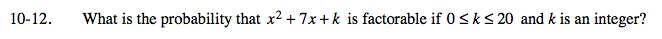### Home > A2C > Chapter Ch10 > Lesson 10.1.1 > Problem10-12

10-12.First list all of the values of k for which the expression is factorable.

x2 + 7x + k is factorable for k = 0, 6, 10, 12.

$\text{The probability is }\frac{4}{21}\approx 19.05.$